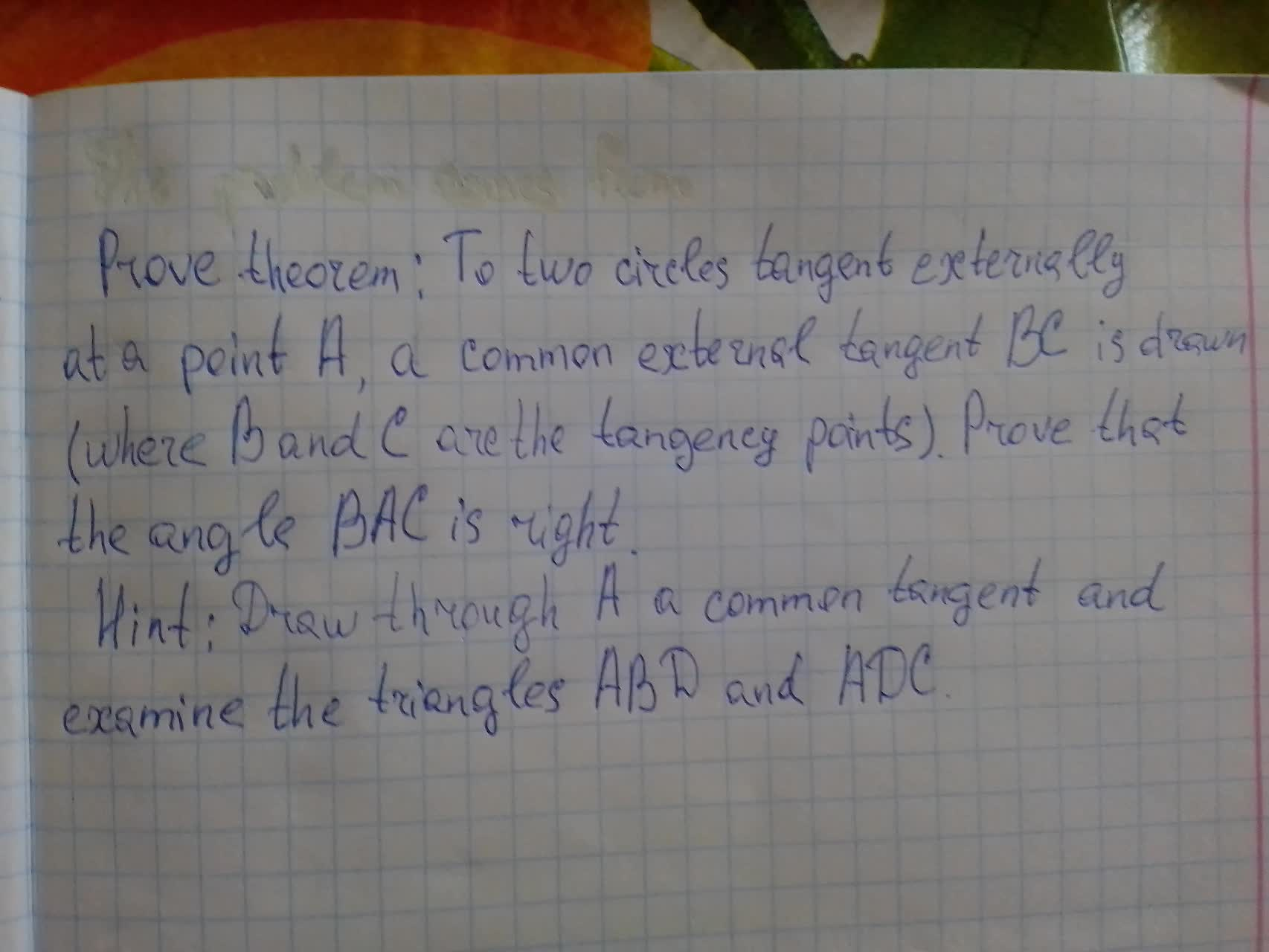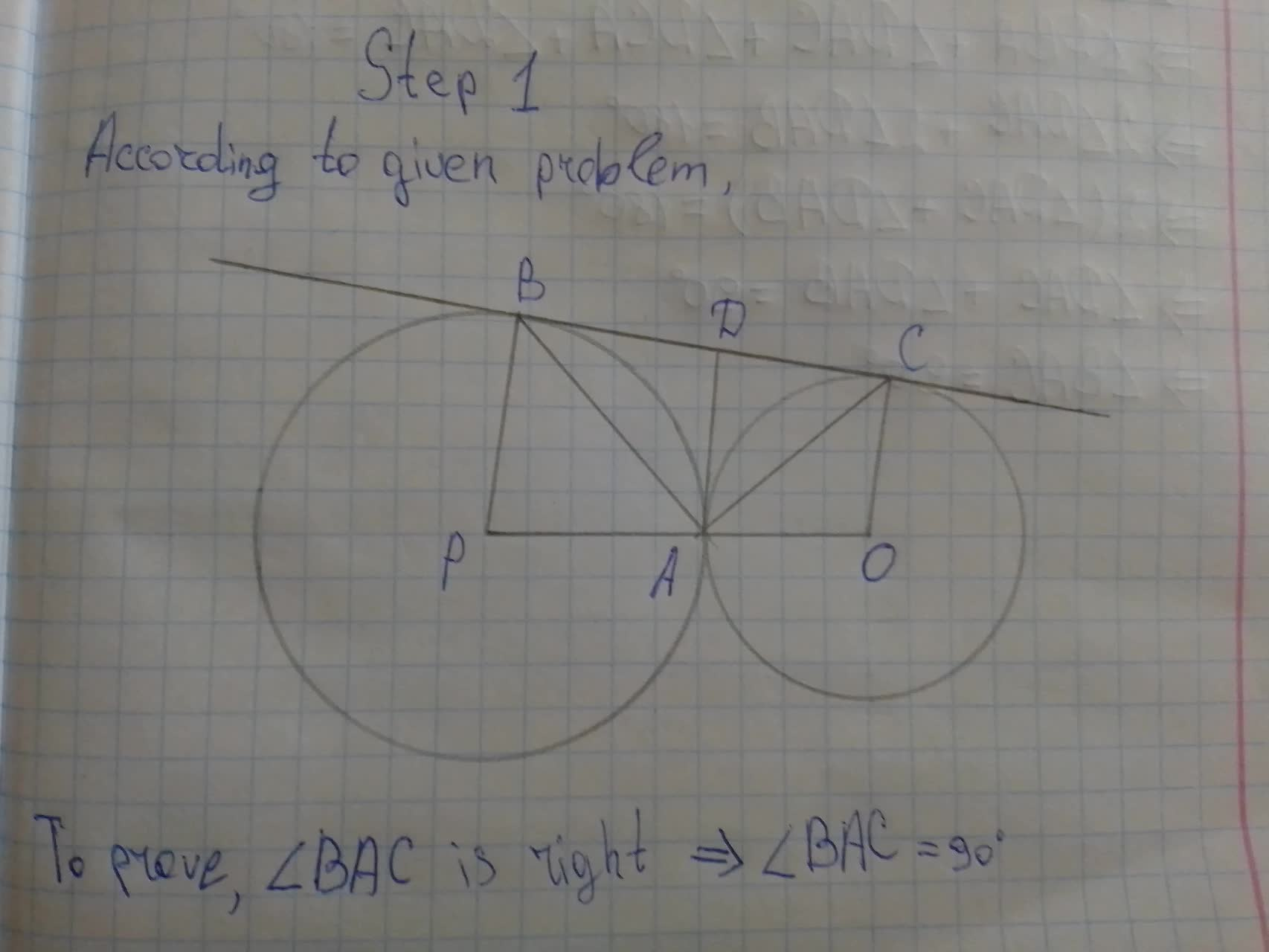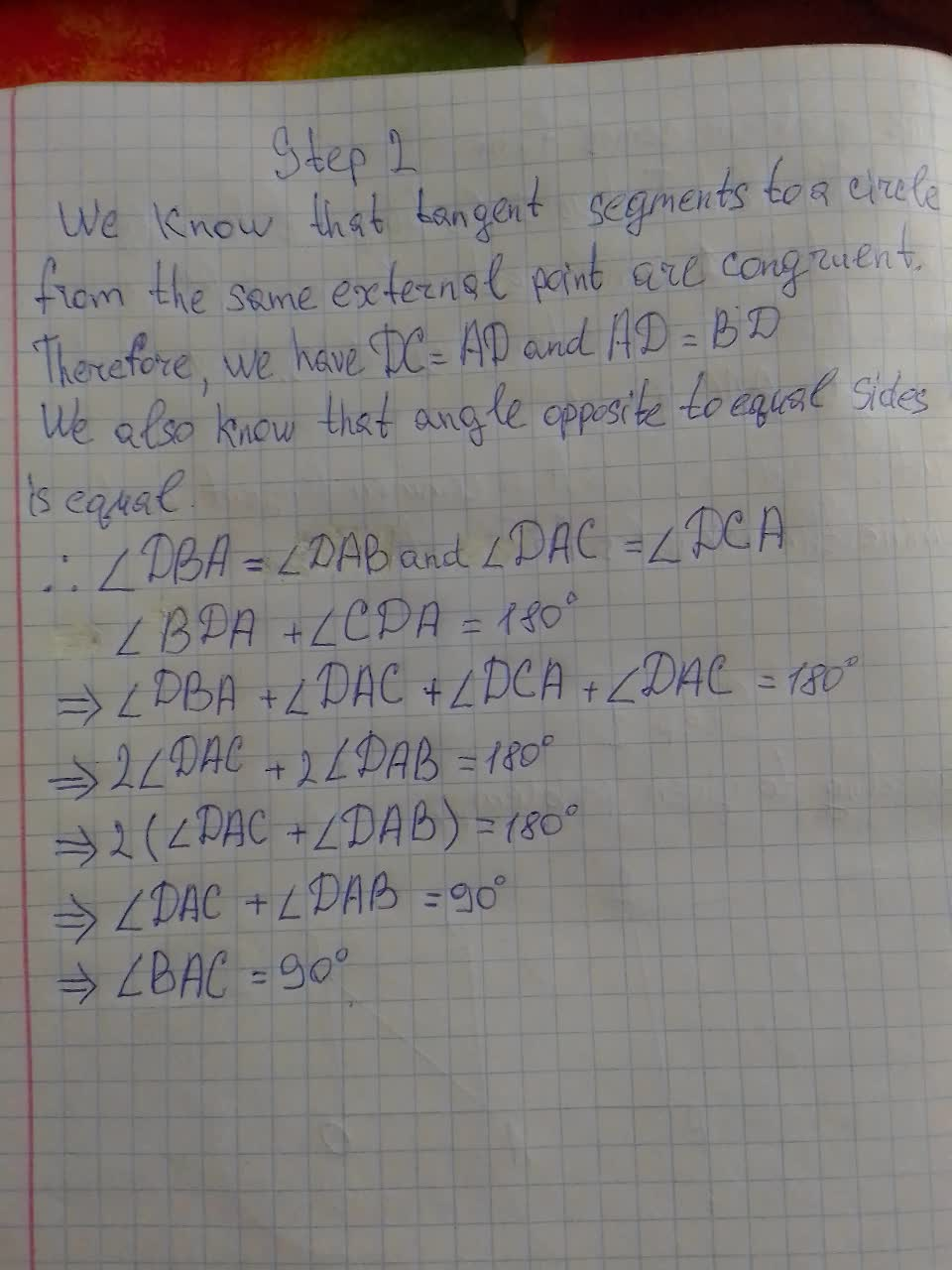Prove theorem: To two circles tangent externally at a point A, a common external tangent BC is drawn(where B and C are the tangency points). Prove that the angle BAC is right.hexacordoK 2021-08-14 Answered
Prove theorem: To two circles tangent externally at a point A, a common external tangent BC is drawn(where B and C are the tangency points). Prove that the angle BAC is right.• Questions are typically answered in as fast as 30 minutes

Solve your problem for the price of one coffee

• Math expert for every subject
• Pay only if we can solve itAlix Ortiz

Prooving the theorem: